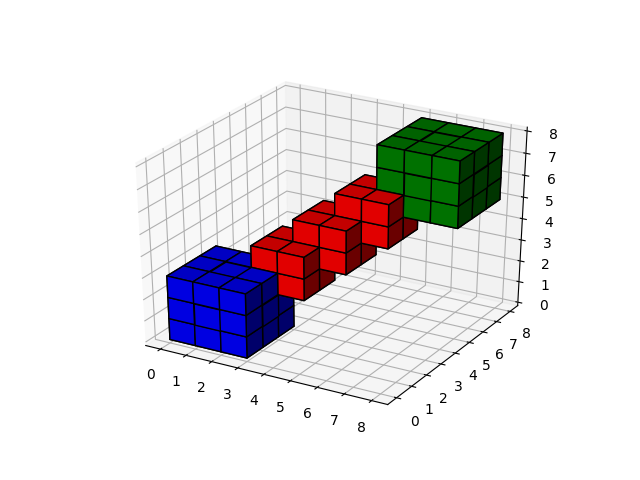# 3D voxel / volumetric plot¶

Demonstrates plotting 3D volumetric objects with ax.voxelsimport matplotlib.pyplot as plt
import numpy as np

# This import registers the 3D projection, but is otherwise unused.
from mpl_toolkits.mplot3d import Axes3D  # noqa: F401 unused import

# prepare some coordinates
x, y, z = np.indices((8, 8, 8))

# draw cuboids in the top left and bottom right corners, and a link between them
cube1 = (x < 3) & (y < 3) & (z < 3)
cube2 = (x >= 5) & (y >= 5) & (z >= 5)
link = abs(x - y) + abs(y - z) + abs(z - x) <= 2

# combine the objects into a single boolean array
voxels = cube1 | cube2 | link

# set the colors of each object
colors = np.empty(voxels.shape, dtype=object)